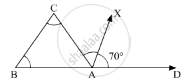Advertisement Remove all ads

# In δAbc, ∠B = ∠C and Ray Ax Bisects the Exterior Angle ∠Dac. If ∠Dax = 70°, Then ∠Acb = - Mathematics

MCQ

In ΔABC, ∠B = ∠C and ray AX bisects the exterior angle ∠DAC. If ∠DAX = 70°, then ∠ACB =

#### Options

• 35°

• 90°

• 70°

• 55°

Advertisement Remove all ads

#### Solution

In the given ΔABC, ∠B = ∠C  . D is the ray extended from point A. AX bisects∠DAC and ∠DAX = 70°

Here, we need to find ∠ACBAs ray AX bisects ∠DAC

∠CAX = ∠DAX = 70°

Thus,

∠DAC = ∠DAX + ∠XAC

= 70° + 70°

= 140°

Now, according to the property, “exterior angle of a triangle is equal to the sum of two opposite interior angles”, we get,

∠DAC = ∠B + ∠C

140° = 2∠C

∠C  =  (140°)/2

= 70°

Thus, ∠ACB = 70°

Is there an error in this question or solution?
Advertisement Remove all ads

#### APPEARS IN

RD Sharma Mathematics for Class 9
Chapter 11 Triangle and its Angles
Q 6 | Page 25
Advertisement Remove all ads
Advertisement Remove all ads
Share
Notifications

View all notifications

Forgot password?
Course